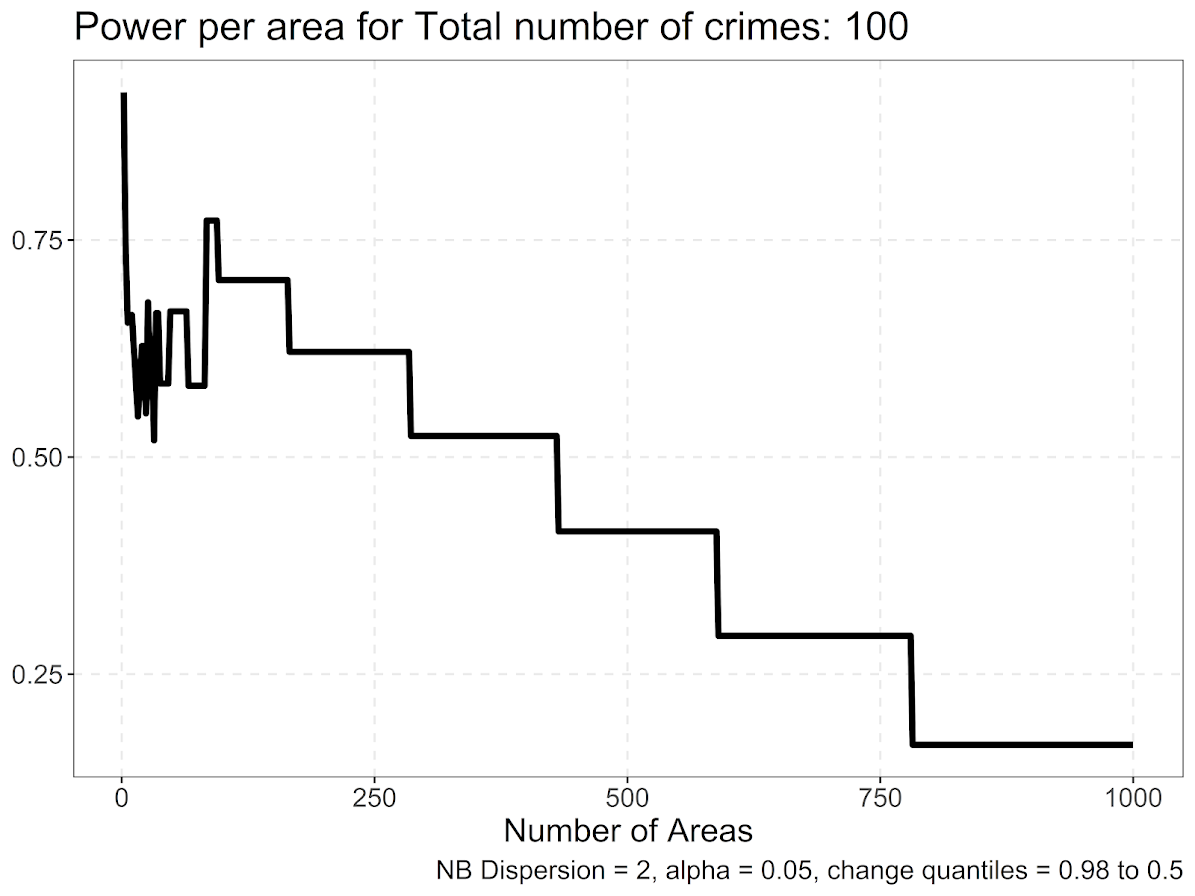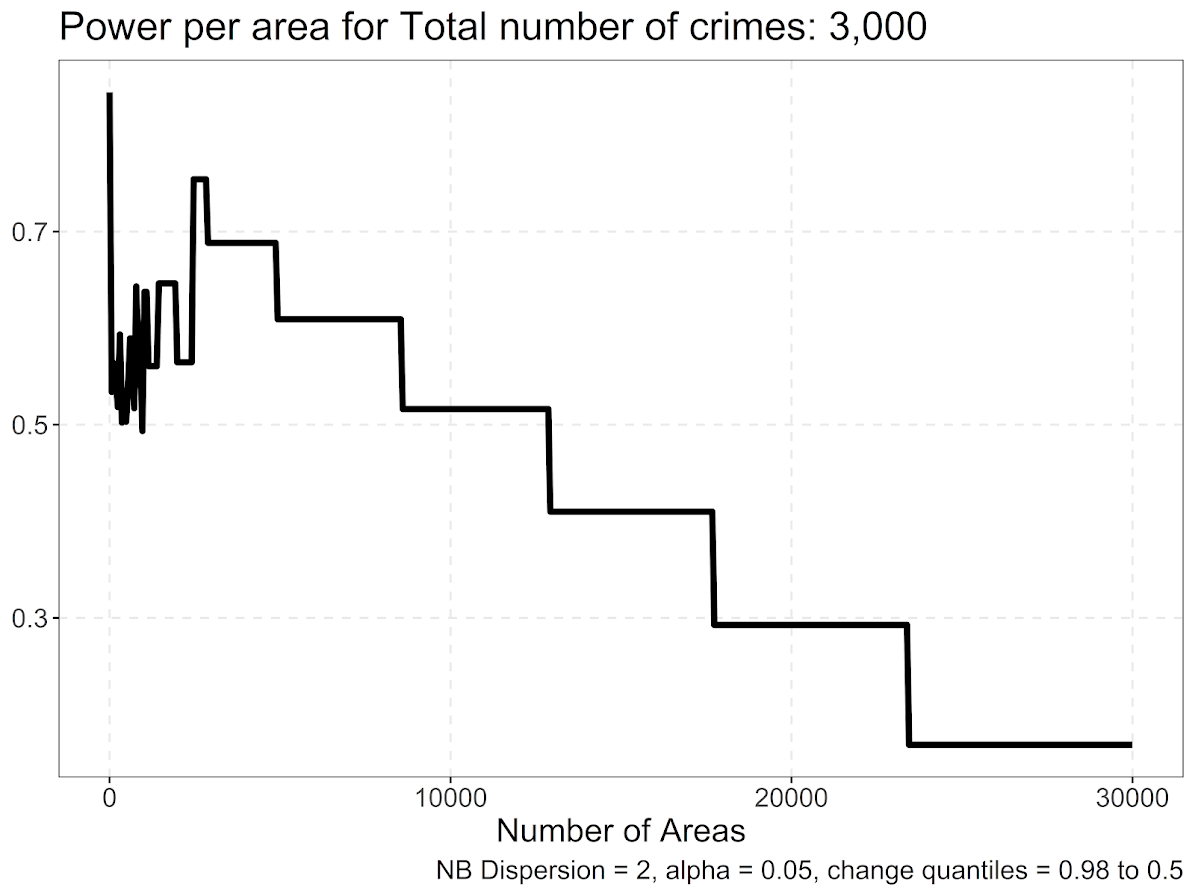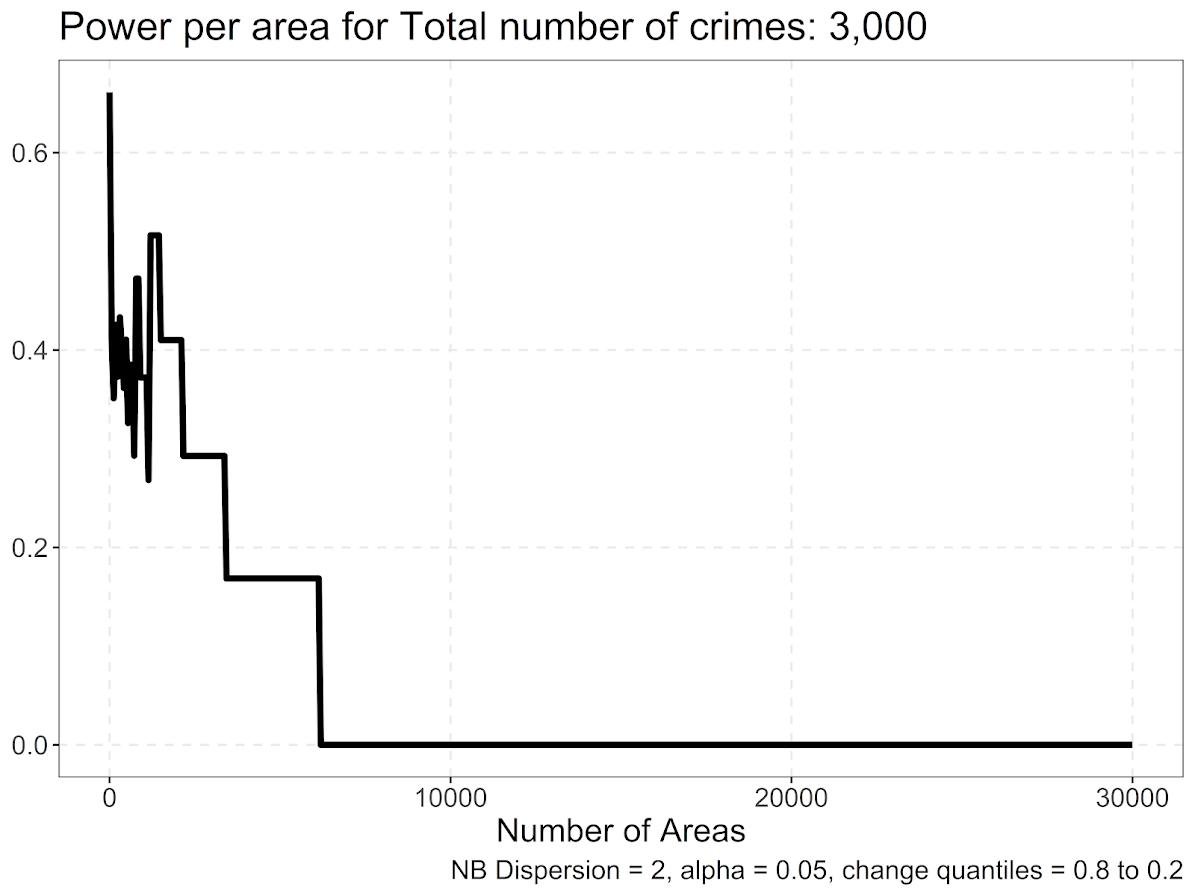# Spatial sample size suggestions for SPPT analysis

I’ve reviewed several papers recently that use Martin Andresen’s Spatial Point Pattern Test (Andresen, 2016). I have been critical of these papers, as I think they are using too small of samples to be reasonable. So here in this blog post I will lay out spatial sample size recommendations. Or more specifically if you have N crimes, advice about how you can conduct the SPPT test in S spatial units of analysis.

Long story short, if you have N crimes, I think you should either use `0.85*N = S` spatial units of analysis at the high end, but can only detect very large changes. To be well powered to detect smaller changes between the two distributions, use `0.45*N = S`. That is, if you have two crime samples you want to compare, and the smaller sample has 1000 crimes, the largest spatial sample size I would recommend is 850 units, but I think 450 units is better.

For those not familiar with the SPPT technique, it compares the proportion of events falling inside a common area (e.g. police beats, census block groups, etc.) between two patterns. So for example in my work I compared the proportion of violent crime and the proportion of SQF in New York City (Wheeler et al., 2018). I think it makes sense as a gross monitoring metric for PDs this way (say for those doing DDACTS, swap out pedestrian stops with traffic stops), so you can say things like `area A had a much lower proportion of crimes than stops, so we should emphasize people do fewer stops in A overall`.

If you are a PD, you may already know the spatial units you want to use for monitoring purposes (say for each police sector or precinct). In that case, you want the power analysis to help guide you for how large a sample you need to effectively know how often you can update the estimates (e.g. you may only have enough traffic stops and violent crimes to do the estimates on a quarterly basis, not a monthly one) Many academic papers though are just generally theory testing, so don’t have an a priori spatial unit of analysis chosen. (But they do have two samples, e.g. a historical sample of 2000 shootings and a current sample of 1000 shootings.) See Martin’s site for a list of prior papers using the SPPT to see it in action.

I’ve reviewed several papers that examine these proportion changes using the SPPT at very tiny spatial units of analysis, such as street segments. They also happen to have very tiny numbers of overall crimes, and then break the crimes into subsequent subsets. For example reviewed a paper that had around 100 crimes in each subset of interest, and had around 20,000 street segments. I totally get wanting to examine micro place crime patterns – but the SPPT is not well suited for this I am afraid.

Ultimately if you chunk up the total number of crimes into smaller and smaller areas, you will have less statistical power to uncover differences. With very tiny total crime counts, you will be basically only identifying differences between areas that go from 0 to 1 or 0 to 2 etc. It also becomes much more important to control for multiple comparisons when using a large number of spatial units. In general this technique is not going to work out well for micro units of analysis, it will only really work out for larger spatial units IMO. But here I will give my best advice about how small you can reasonably go for the analysis.

# Power analysis logic

There are quite a few different ways people have suggested to determine the spatial sample for areas when conducting quadrat analysis (e.g. when you make your own spatial areas). So one rule of thumb is to use `2*A/N`, where A is the area of the study and N is the total number of events (Paez, 2021).

Using the SPPT test itself, Malleson et al. (2019) identify the area at which the spatial pattern exhibits the highest similarity index with itself using a resampling approach. Ramos et al. (2021) look at the smallest spatial unit at which the crime patterns within that unit show spatial randomness.

So those later two take an error metric based approach (the spatial unit of analysis likely to result in the miminal amount of error, with error defined different ways). I take a different approach here – power analysis. We want to compare to spatial point patterns for proportional differences, how can we construct the test to be reasonably powered to identify differences we want to detect?

I do not have a perfect way to do this power analysis, but here is my logic. Crime patterns are often slightly overdispersed, so here I assume if you split up say 1000 crimes into 600 areas, it will have an NB2 distribution with a mean of `1000/600 = 1.67` and an overdispersion parameter of 2. (I assume this parameter to be 2 for various reasons, based on prior analysis of crime patterns, and that 2 tends to be in the general ballpark for the amount of overdispersion.) So now we want to see what it would take to go from a hot spot of crime, say the 98th percentile of this distribution to the median 50th percentile.

So in R code, to translate the NB2 mean/dispersion to N & P notation results in N & P parameters of `1.0416667` and `0.3846154` respectively:

``````trans_np <- function(mu,disp){
a <- disp
x <- mu^2/(1 - mu + a*mu)
p <- x/(x + mu)
n <- (mu*p)/(1-p)
return(c(n,p))
}

# Mean 1000/600 and dispersion of 2
nb_dis <- trans_np(1000/600,2)``````

Now we want to see what the counts are to go from the 98th to the 50th percentile of this distribution:

``crime_counts <- qnbinom(c(0.98,0.5), size=nb_dis, prob=nb_dis)``

And this gives us a result of ` 8 1` in the `crime_counts` object. So a hot spot place in this scenario will have around 8 crimes, and the median will be around 1 crime in our hypothetical areas. So we can translate these to percentages, and then feed them into R’s `power.prop.test` function:

``````crime_prop <- crime_counts/1000
power.prop.test(n = 1000, p1 = crime_prop, p2 = crime_prop)``````

And this gives us a result of:

``````     Two-sample comparison of proportions power calculation

n = 1000
p1 = 0.008
p2 = 0.001
sig.level = 0.05
power = 0.6477139
alternative = two.sided

NOTE: n is number in *each* group``````

Note that this is for one N estimate, and assumes that N will be the same for each proportion. In practice for the SPPT test this is not true, oftentimes we have two crime samples (or crime vs police actions like stops), which have very different total baseline N’s. (It is part of the reason the test is useful, it doesn’t make so much sense in that case to compare densities as it does proportions.) So subsequently when we do these estimates, we should either take the average of the total number of crimes we have in our two point patterns for SPPT (if they are close to the same size), or the minimum number of events if they are very disparate. So if you have in sample A 1000 crimes, and sample B 2000 crimes, I think you should treat the N in this scenario as 1500. If you have 5000 crimes vs 1000000 crimes, you should treat N here as 5000.

So that estimate above is for one set of crimes (1000), and one set of areas (600). But what if we vary the number of areas? At what number of areas do we have the maximum power?

So I provide functions below to generate the power estimate curve, given these assumptions about the underlying crime distribution (which will generally be in the ballpark for many crime patterns, but not perfect), for varying numbers of spatial units. Typically we know the total number of crimes, so we are saying given I have N crimes, how finely can a split them up to check for differences with the SPPT test.

Both the Malleson and Ramos article place their recommendations in terms of area instead of total number of units. But it would not surprise me if our different procedures end up resulting in similar recommendations based on the observed outputs of each of the papers. (The `2A/N` quadrat analysis suggestion translates to `N*0.5` total number of areas, pretty close to my `0.45*N` suggestion for example.)

# R Code

Below I have a nicer function to do the analysis I walked through above, but give a nice power curve and dataframe over various potential spatial sample sizes:

``````# SPPT Power analysis example
library(ggplot)

# See https://andrewpwheeler.com/2015/01/03/translating-between-the-dispersion-term-in-a-negative-binomial-regression-and-random-variables-in-spss/
trans_np <- function(mu,disp){
a <- disp
x <- mu^2/(1 - mu + a*mu)
p <- x/(x + mu)
n <- (mu*p)/(1-p)
return(c(n,p))
}

diff_suggest <- function(total_crimes,areas=round(seq(2,total_crimes*10,length.out=500)),
change_quant=c(0.98,0.5), nb_disp=2, alpha = 0.05,
plot=TRUE){
# Figure out mean
areas <- unique(areas)
mean_cr <- total_crimes/areas
# Initialize some vectors to place the results
n_areas <- length(areas)
power <- vector("numeric",length=n_areas)
hign <- power
lown <- power
higp <- power
lowp <- power
# loop over areas and calculate power
for (i in 1:length(areas)){
# Negative binomial parameters
dp <- trans_np(mean_cr[i],nb_disp)
hilo <- qnbinom(change_quant, size=dp, prob=dp)
hilo_prop <- hilo/total_crimes
# Power for test
pow <- power.prop.test(n = total_crimes, p1 = hilo_prop, p2 = hilo_prop,
sig.level = alpha)
# Stuffing results in vector
power[i] <- pow\$power
hign[i] <- hilo
lown[i] <- hilo
higp[i] <- hilo_prop
lowp[i] <- hilo_prop
}
Ncrimes <- rep(total_crimes,n_areas)
res_df <- data.frame(Ncrimes,areas,mean_cr,power,hign,lown,higp,lowp)
# replacing missing with 0
res_df[is.na(res_df)] <- 0
if (plot) {
require(ggplot2)
fmt_cr <- formatC(total_crimes, format="f", big.mark=",", digits=0)
title_str <- paste0("Power per area for Total number of crimes: ",fmt_cr)
cap_str <- paste0("NB Dispersion = ",nb_disp,", alpha = ",alpha,
", change quantiles = ",change_quant," to ",change_quant)
p <- ggplot(data=res_df,aes(x=areas,y=power)) + geom_line(size=1.5) +
theme_bw() + theme(panel.grid.major = element_line(linetype="dashed")) +
labs(x='Number of Areas',y=NULL,title=title_str,caption=cap_str) +
scale_x_continuous(minor_breaks=NULL) + scale_y_continuous(minor_breaks=NULL) +
theme(text = element_text(size=16), axis.title.y=element_text(margin=margin(0,10,0,0)))
print(p)
}
return(res_df)
}``````

Once that function is defined, you can make a simple call like below, and it gives you a nice graph of the power given different numbers of grid cells:

``diff_suggest(100)``So you can see here we never have very high power over this set of parameters. It is also non-monotonic and volatile at very small numbers of spatial units of analysis (at which the overdispersion assumption likely does not hold, and probably is not of much interest). But once that volatility tamps down we have stepped curve, that ends up happening to step whenever the original NB distribution changes from particular integer values.

So what happens with the power curve if we up the number of crimes to 3000?

``diff_suggest(3000)``So those two patterns are quite similar. It happens that when breaking down to the smaller units, the highest power scenario is when the crimes are subdivided into around 0.85 fewer spatial units than total crimes. So if you have 1000 crimes, in this scenario I would suggest to use 850 areas.

Also note the behavior when you break it down into a very large number of spatial units S, where `S >> N`, you get a progressive decline until around 0 power in this analysis. E.g. if you have 100 times more spatial units than observations, only a handful of locations have any crimes, and the rest are all 0’s. So you need to be able to tell the difference between 1/N and 0, which is tough (and any inferences you do make will just pretty much be indistinguishable from noise).

What about if we change the quantiles we are examining, and instead of looking at the very high crime place to the median, look at the 80th percentile to the 20th percentile:

``diff_suggest(3000, change_quant=c(0.8,0.2))``We have a similar step pattern, but here the power is never as high as before, and only maxes out slightly above 0.5. It happens that this 0.5 power is around `number of crimes*0.45`. So this suggests that to uncover more middling transitions, one would need to have less than half the number of spatial units of crime observed. E.g. if you have 1000 crimes, I would not suggest any more than 450 spatial units of analysis.

So the first scenario, `crimes*0.85` you could say something like this is the highest power scenario to detect changes from very high crime locations (aka hot spots), to the middle of the distribution. For the second scenario (my preferred offhand), is to say `crimes*0.45` total spatial units results in the highest power scenario to detect more mild changes in the middle of the distribution (and detecting changes from hot spots to cold spots thus have even more power).

For now that is the best advice I can give for determining the spatial sample size for the SPPT test. Will have a follow up blog post on using R to make a grid to conduct the test.

Also I have been wondering about the best way to quantify changes in the overall ranking. I have not come upon a great solution I am happy with though, so will need to think about it some more.